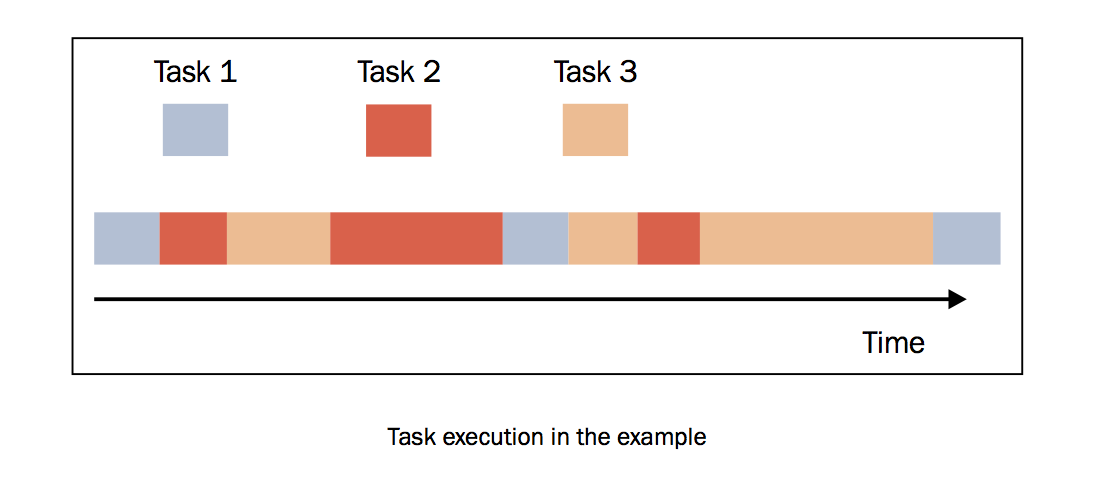# 3. 使用Asyncio管理事件循环¶

Python的Asyncio模块提供了管理事件、协程、任务和线程的方法，以及编写并发代码的原语。此模块的主要组件和概念包括：

• 事件循环: 在Asyncio模块中，每一个进程都有一个事件循环。
• 协程: 这是子程序的泛化概念。协程可以在执行期间暂停，这样就可以等待外部的处理（例如IO）完成之后，从之前暂停的地方恢复执行。
• Futures: 定义了 `Future` 对象，和 `concurrent.futures` 模块一样，表示尚未完成的计算。

## 3.1. 什么是事件循环¶

```while (1) {
events = getEvents();
for (e in events)
processEvent(e);
}
```

## 3.2. 准备工作¶

Asyncio提供了一下方法来管理事件循环：

• `loop = get_event_loop()`: 得到当前上下文的事件循环。
• `loop.call_later(time_delay, callback, argument)`: 延后 `time_delay` 秒再执行 `callback` 方法。
• `loop.call_soon(callback, argument)`: 尽可能快调用 `callback`, `call_soon()` 函数结束，主线程回到事件循环之后就会马上调用 `callback`
• `loop.time()`: 以float类型返回当前时间循环的内部时间。
• `asyncio.set_event_loop()`: 为当前上下文设置事件循环。
• `asyncio.new_event_loop()`: 根据此策略创建一个新的时间循环并返回。
• `loop.run_forever()`: 在调用 `stop()` 之前将一直运行。

## 3.3. 如何做…¶

```import asyncio
import datetime
import time

def function_1(end_time, loop):
print ("function_1 called")
if (loop.time() + 1.0) < end_time:
loop.call_later(1, function_2, end_time, loop)
else:
loop.stop()

def function_2(end_time, loop):
print ("function_2 called ")
if (loop.time() + 1.0) < end_time:
loop.call_later(1, function_3, end_time, loop)
else:
loop.stop()

def function_3(end_time, loop):
print ("function_3 called")
if (loop.time() + 1.0) < end_time:
loop.call_later(1, function_1, end_time, loop)
else:
loop.stop()

def function_4(end_time, loop):
print ("function_5 called")
if (loop.time() + 1.0) < end_time:
loop.call_later(1, function_4, end_time, loop)
else:
loop.stop()

loop = asyncio.get_event_loop()

end_loop = loop.time() + 9.0
loop.call_soon(function_1, end_loop, loop)
# loop.call_soon(function_4, end_loop, loop)
loop.run_forever()
loop.close()
```

```python3 event.py
function_1 called
function_2 called
function_3 called
function_1 called
function_2 called
function_3 called
function_1 called
function_2 called
function_3 called
```

## 3.4. 讨论¶```loop = asyncio.get_event_loop()
```

```end_loop = loop.time() + 9.0
loop.call_soon(function_1, end_loop, loop)
```

```def function_1(end_time, loop):
print ("function_1 called")
if (loop.time() + 1.0) < end_time:
loop.call_later(1, function_2, end_time, loop)
else:
loop.stop()
```

• `end_time`: 定义了 `function_1()` 可以运行的最长时间，并通过 `call_later` 方法传入到 `function_2()` 中作为参数
• `loop`: 之前通过 `get_event_loop()` 方法得到的事件循环

`function_1()` 的任务非常简单，只是打印出函数名字。当然，里面也可以写非常复杂的操作。

```print ("function_1 called")
```

```if (loop.time() + 1.0) < end_time:
loop.call_later(1, function_2, end_time, loop)
else:
loop.stop()
```

`function_2()``function_3()` 的作用类似。

```loop.run_forever()
loop.close()
```